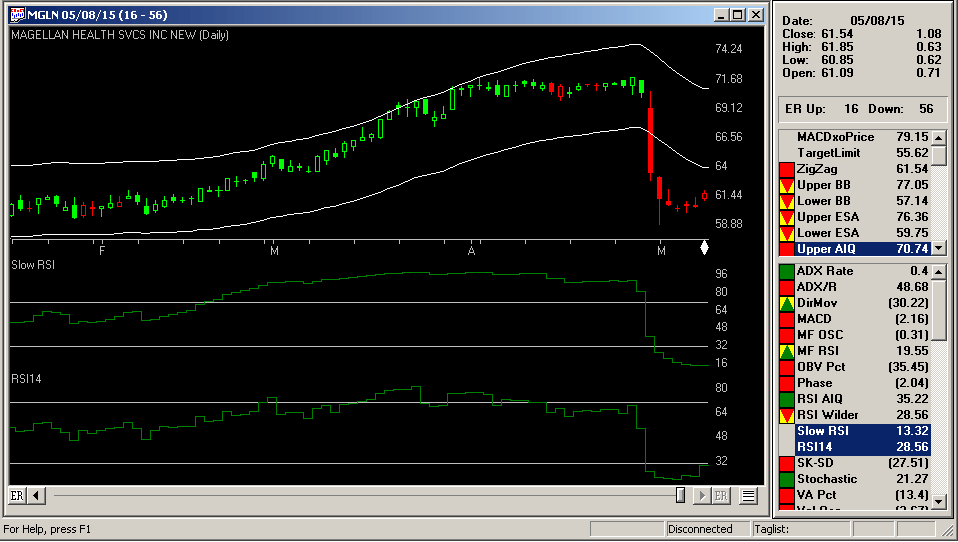# The Slow Relative Strength Index

The AIQ code based on Vitali Apirine’s article in STOCKS & COMMODITIES, “The Slow Relative Strength Index,” is shown here. This code for the slow RSI (SRSI) is for use as an indicator. A sample chart illustrating the SRSI is shown in Figure 7.FIGURE 7: AIQ. This example chart shows the slow RSI (6,14) compared to the classic RSI (14).
```!THE SLOW RELATIVE STRENGTH INDEX
!Author: Vitali Aprine, TASC April 2015
!Coded by: Richard Denning 5/3/2015

!INPUTS FOR INDICATOR:
emaLen is 6.
wilderLen is 14.

!INDICATOR FORMULAS:
ema is expavg([close],emaLen).
pDif is iff([close] - ema > 0,[close] - ema,0).
nDif is iff([close] - ema < 0,ema - [close],0).

rsiLen is 2 * wilderLen - 1.
AvgU  is expavg(pDif,rsiLen).
AvgD  is expavg(nDif,rsiLen).
srsi is 100-(100/(1+(AvgU/AvgD))). !PLOT
```